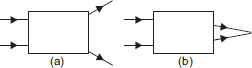# User Forum

Subject :NSO    Class : Class 10

In the following figures, optical instruments are placed inside boxes (A) and (B). Which of the following options is correct for these?A In (A), the incident rays are diverged after refractions, so lens is concave.
B In (B), the incident rays are converged after refraction so mirror is convex.
C In (A), the incident rays are diverged after refraction so mirror is convex.
D In (B), the incident rays are converged after refraction so lens is concave.

Aren't both options A and B correct?